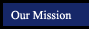Algebra 1 - Concepts, Skills and Problem Solving
Solving Linear Equations
Chapter 2
 Section Title Tutorials from this book More Examples (in pop-up window) 2.1 p.70 Writing Equations new per4 per6 notebook express 2.2 p.78 Solving Equations by Using Addition and Subtration new per4 per6 notebook express Practice Game -Solving 1-Step Equations Using Addition and Subtraction Practice Game -Solving 1-Step Equations Using Addition and Subtraction (With a Fraction!) Practice Game -Solving 1-Step Equations Using Addition and Subtraction (With 2 Fractions!) 2.3 p.85 Solving Equations by Using Multiplication and Division new per4 per6 notebook express Practice Game -Solving 1-Step Equations Using Multiplication and Division Practice Game - Solving 1-Step Equations with Fractions Next to the Variable 1 Step Equation Practice Activiity (random mix of addition, subtraction, multiplication and division) 2.4 p.92 Solving Mult-Step Equations new per4 per6 notebook express 2 Step Equation Practice Game - Level 0 2 Step Equation Practice Game - Level 1 2.5 p.98 Solving Equations with the Variable on Each Side new per4 per6 notebook express Practice Game - Equations With Variables on Both Sides - Level 0 Practice Game - Equations With Variables on Both Sides - Level 1 Practice Game - Equations With Variables on Both Sides - Level 2 Practice Game - Equations With Variables on Both Sides (and Parentheses!) Practice Game - Equations With Variables and Parentheses on Both Sides - Level 0 Practice Game - Equations With Variables and Parentheses on Both Sides - Level 1 2.6 p.105 Ratios and Proportions new per4 per6 notebook express Practice Game - Turn Proportions into Regular Equations 2.7 p.111 Percent of Change new per4 per6 notebook express 2.8 p.117 Solving for a Specific Variable new per4 per6 notebook express 2.9 p.122 Weighted Averages

Online Scientific Calculator from Calculator.com

Math Glossary
(more sophisticated than it looks!)